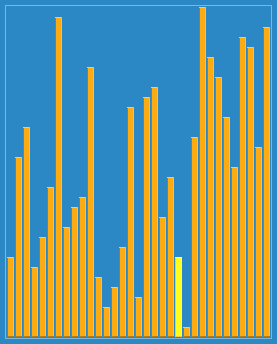# Shell Sort Algorithm

Data Structures and AlgorithmsDonald L. Shell- the man behind shell sort

About Shell Sort and its History

Donald L. Shell published the shell sort in the year 1959. The shell sort algorithm is an extension and improved version of the insertion sort algorithm. Donald L. Shell noticed a problem with the insertion sort algorithm and tried to fix it in his shell sort algorithm. In the case of the insertion sort algorithm, he noticed that he had to move a lot of elements to make places for the new elements to be inserted in the sorted part of the array, and for large arrays, this will require a lot of time.Visual representation of Shell sort

The idea behind shell sort is that we compare elements that are distant apart rather than at adjacent positions and try to shift the smaller values on the left side and larger values on the right side. So, if there are N elements then it starts with a value “gap” < N. As this algorithm is an improved version of insertion sort, it uses insertion sorting as well. In each iteration, it keeps on reducing the value of the gap until it reaches the last iteration when the gap becomes 1. If the gap is set differently, the time complexity will be different.

Note: Shell sort is a non-stable sorting algorithm since the elements with similar values can change the order of their sequences.

Here’s a step by step instructions on how to apply shell sort to a list:

Step 1: Divide the list into n/2 sublists.

Step 2: Sort each sublist using insertion sort.

Step 3: Merge the sublists.

Step 4: Halve the number of sublists.

Step 5: Repeat steps 2 and 3 until the number of sublists becomes “1”.

# Let’s take an example to understand:

Consider the following unsorted elements.

X = [18, 32, 12, 5, 38, 33, 16, 2]

No. of elements = 8

gap = floor(N/2) = floor(8/2) = 4

There are 8 elements in the list, so in the first iteration, we will divide the list into (8/2) = 4 sublists. Sublist 1 contains the 1st and 5th elements(18, 38), sublist 2 contains the 2nd and 6th elements (32, 33), and so on.

Step 1) Now, perform insertion sort on sublist 1. As there are only 2 elements in sublist 1, so just one comparison will be needed.

18 is smaller than 38, so they will not swap.

Now, we will perform insertion sorts on each of the other sublists in the same way.

Step 2) In sublist 2 ( 32, 33)

32 is smaller than 33, so they will not swap.

Step 3) In sublist 3 ( 12, 16)

12 is smaller than 16, so they will not swap.

Step 4) In sublist 4 (5, 2)

5 is larger than 2, so the elements are swapped.

Resulting numbers after increment 4 pass:

X = [ 18, 32, 12, 2, 38, 33, 16, 5 ]

gap = floor(4/2) = 2

Increment 2: Sublist 1(18, 12, 38, 16), Sublist 2(32, 2, 33, 5)

Perform insertion sort on sublist 1 and sort them in their appropriate location:

X =[12, 38, 16, 2, 18, 33, 38, 5]

Now, perform insertion sort on sublist 2

X = [12, 2, 16, 5, 18, 32, 38, 33]

The last increment of Shell sort is basically an insertion sort algorithm.

gap = floor(2/2) = 1

Sublist 1:(12, 2, 16, 5, 18, 32, 38, 33)

After sorting,

X = [ 2, 5, 12, 16, 18, 32, 33, 38]

Finally, the array is sorted.

# Pseudocode

`procedure ShellSort()   arr: Array    /* calculate gap*/   while gap< arr.length /3 do:      gap= gap * 3 + 1        end while      while gap> 0 do:      for outer = gap; outer < arr.length; outer ++ do:      /*choosing value to be inserted */      valueToInsert = arr[outer]      inner = outer;         /*shift element to right*/         while inner > gap -1 && arr[inner - gap] >= valueToInsert do:            arr[inner]=arr[inner-interval]            inner = inner-gap         end while      /* inserting num at hole position */      arr[inner] = valueToInsert      end for   /* calculate gap*/   gap=(gap-1)/3;      end while   end procedure`

# Implementation of shell sort in c++

`#include  <iostream>using namespace std;// shellsort implementationint shellSort(int arr[], int N){for (int gap = N/2; gap > 0; gap /= 2){for (int i = gap; i < N; i += 1){//sort sub lists created by applying gapint temp = arr[i];int j;for (j = i; j >= gap && arr[j - gap] > temp; j -= gap)arr[j] = arr[j - gap];arr[j] = temp;}}return 0;}int main(){int arr[] = {18, 32, 12, 5, 38, 33, 16, 2}, i;//Calculate size of arrayint N = sizeof(arr)/sizeof(arr);cout << "Array to be sorted: \n";for (int i=0; i<N; i++)cout << arr[i] << " ";shellSort(arr, N);cout << "\nArray after shell sort: \n";for (int i=0; i<N; i++)cout << arr[i] << " ";return 0;}`

# Big O complexity for Shell sort:

Worst-case performance: O(n²)

Best-case performance: O(n*log n)

# Space complexity:

Auxiliary space: O(1).
Total space: O(n).

# Applications of Shell sort:

• The C standard library uses shell sort, instead of qsort, when dealing with embedded systems.
• Compressors, such as bzip2, also use it to avoid problems that could come when sorting algorithms exceed a language’s recursion depth.
• It is also used in the Linux kernel because it does not use the call stack.

# Advantages — Shell Sort

• It is only efficient for the smaller number of elements.
• It is faster than the bubble sort algorithm.

# Disadvantages — Shell Sort

• It is complex in structure and a bit more difficult to understand.
• The shell sort algorithm is slower than the merge sort, quick sort, and heap sort algorithms.

# Conclusion

Shell sort is a highly efficient algorithm that performs better than Insertion sort by taking fewer moves to sort the list but it has a bigger cache miss ratio than Quick Sort. It improves the efficiency of insertion sort by quickly shifting elements to their destination.